29Jun, 2020
Language blog :
English
Share blog :
29 June, 2020
English

# Top 10 Machine Learning Algorithms for ML Beginners

ByIn the last decade machine learning becomes one of the hottest topics in the world, Andrew Ng considers it as the new electricity. In today’s world machine learning powers many of the services we use — recommendation systems like those on Netflix, YouTube, and Spotify; search engines like Google and Baidu; social-media feeds like Facebook and Twitter; voice assistants like Siri and Alexa. The list goes on.

Having known that, let’s see how machine learning works. In simple terms, machine learning algorithms use statistics to find patterns in massive amounts of data. The data are also known as the dataset, it’s could contain images, texts, words, and clicks. Each dataset contains several features, taking Netflix’s recommendation system as an example; these features could be the movie rating, genre, language, length, and so on. Then Netflix runs its machine learning model on this data and outputs a list of recommended movies to the user.

In this blog article, I will cover the most used machine learning algorithms.

## Linear Regression

In machine learning, we have a set of input variables (x) that are used to determine an output variable (y). A relationship exists between the input variables and the output variable. The goal of ML is to quantify this relationship.

In Linear Regression, the relationship between the input variables (x) and output variable (y) is expressed as an equation of the form y = a + bx. Thus, the goal of linear regression is to find out the values of coefficients a and b. Here, a is the intercept and b is the slope of the line.

## Logistic Regression

Logistic regression is limited to linear regression with non-linearity (sigmoid function or tanh is mainly used) after applying weights, therefore, the output limit is close to + / — classes (which equals 1 and 0 in the case of sigmoid). Cross-entropy loss functions are optimized using the gradient descent method.

Note for beginners: logistic regression is used for classification, not regression. In general, it is similar to a single-layer neural network. Learned using optimization techniques such as gradient descent or L-BFGS. NLP developers often use it, calling it “the maximum entropy classification method”.

This is what a sigmoid looks like: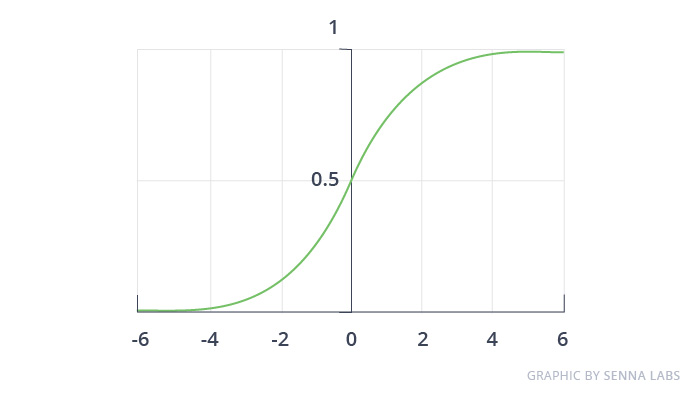## Linear Discriminant Analysis (LDA)

Logistic Regression is a classification algorithm traditionally limited to only two-class classification problems. If you have more than two classes then the Linear Discriminant Analysis algorithm is the preferred linear classification technique.

The representation of LDA is pretty straight forward. It consists of the statistical properties of your data, calculated for each class. For a single input variable this includes:

1. The mean value for each class
2. The variance calculated across all classesPredictions are made by calculating a discriminant value for each class and making a prediction for the class with the largest value. The technique assumes that the data has a Gaussian distribution (bell curve), so it is a good idea to remove outliers from your data beforehand. It’s a simple and powerful method for classification predictive modeling problems.

## Decision Trees

One of the most common machine learning algorithms. Used in statistics and data analysis for predictive models. The structure represents the “leaves” and “branches”. Attributes of the objective function depend on the “branches” of the decision tree, the values of the objective function are recorded in the “leaves”, and the remaining nodes contain attributes for which the cases differ.

## K-Means Clustering

Everyone’s favorite uncontrolled clustering algorithm. But, let’s clarify what clustering is:

Clustering (or cluster analysis) is the task of breaking up a set of objects into groups called clusters. Inside each group, there should be “similar” objects, and the objects of different groups should be as different as possible. The main difference between clustering and classification is that the list of groups is not clearly defined and is determined during the operation of the algorithm.

The k-means algorithm is the simplest, but at the same time, rather an inaccurate clustering method in the classical implementation. It splits the set of elements of a vector space into a previously known number of clusters k.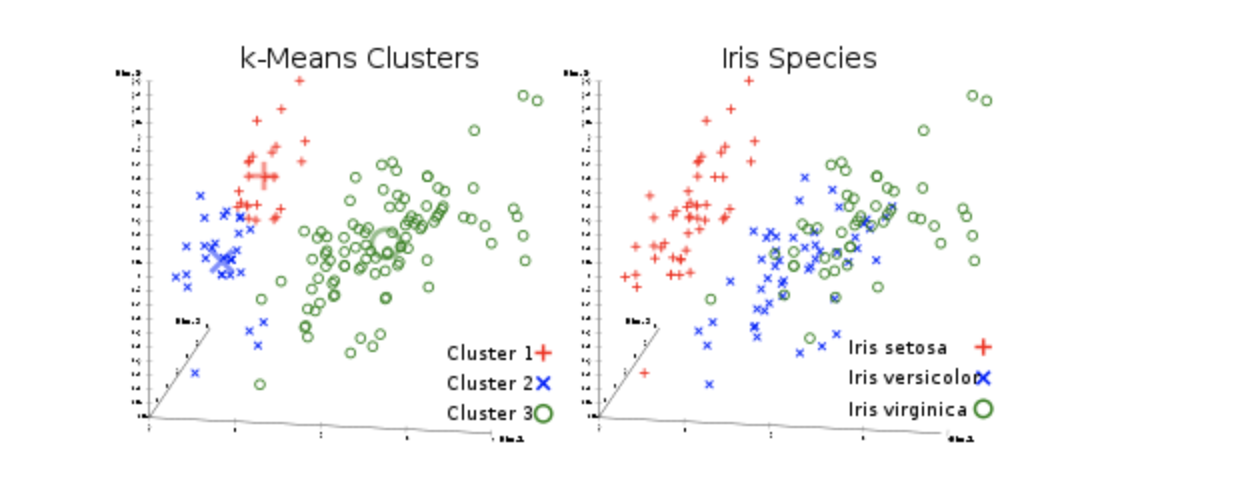## Naive Bayes

Naive Bayes is a simple but surprisingly powerful algorithm for predictive modeling.

The model is comprised of two types of probabilities that can be calculated directly from your training data: 1) The probability of each class; and 2) The conditional probability for each class given each x value. Once calculated, the probability model can be used to make predictions for new data using Bayes Theorem. When your data is real-valued it is common to assume a Gaussian distribution (bell curve) so that you can easily estimate these probabilities.

Naive Bayes is called naive because it assumes that each input variable is independent. This is a strong assumption and unrealistic for real data, nevertheless, the technique is very effective on a large range of complex problems.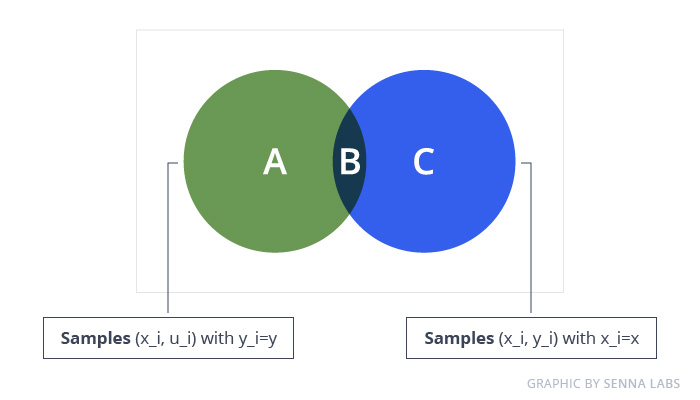## K-Nearest Neighbours

The KNN algorithm is very simple and very effective. The model representation for KNN is the entire training dataset. Simple right?

Predictions are made for a new data point by searching through the entire training set for the K most similar instances (the neighbors) and summarizing the output variable for those K instances. For regression problems, this might be the mean output variable, for classification problems this might be the mode (or most common) class value.

The trick is in how to determine the similarity between the data instances. The simplest technique if your attributes are all of the same scale (all in inches for example) is to use the Euclidean distance, a number you can calculate directly based on the differences between each input variable.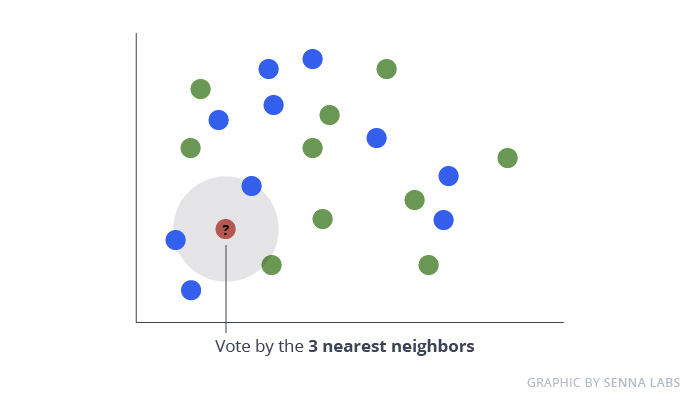## Support Vector Machines

Support Vector Machines are perhaps one of the most popular and talked about machine learning algorithms.

A hyperplane is a line that splits the input variable space. In SVM, a hyperplane is selected to best separate the points in the input variable space by their class, either class 0 or class 1. In two-dimensions, you can visualize this as a line, and let’s assume that all of our input points can be completely separated by this line. The SVM learning algorithm finds the coefficients that result in the best separation of the classes by the hyperplane.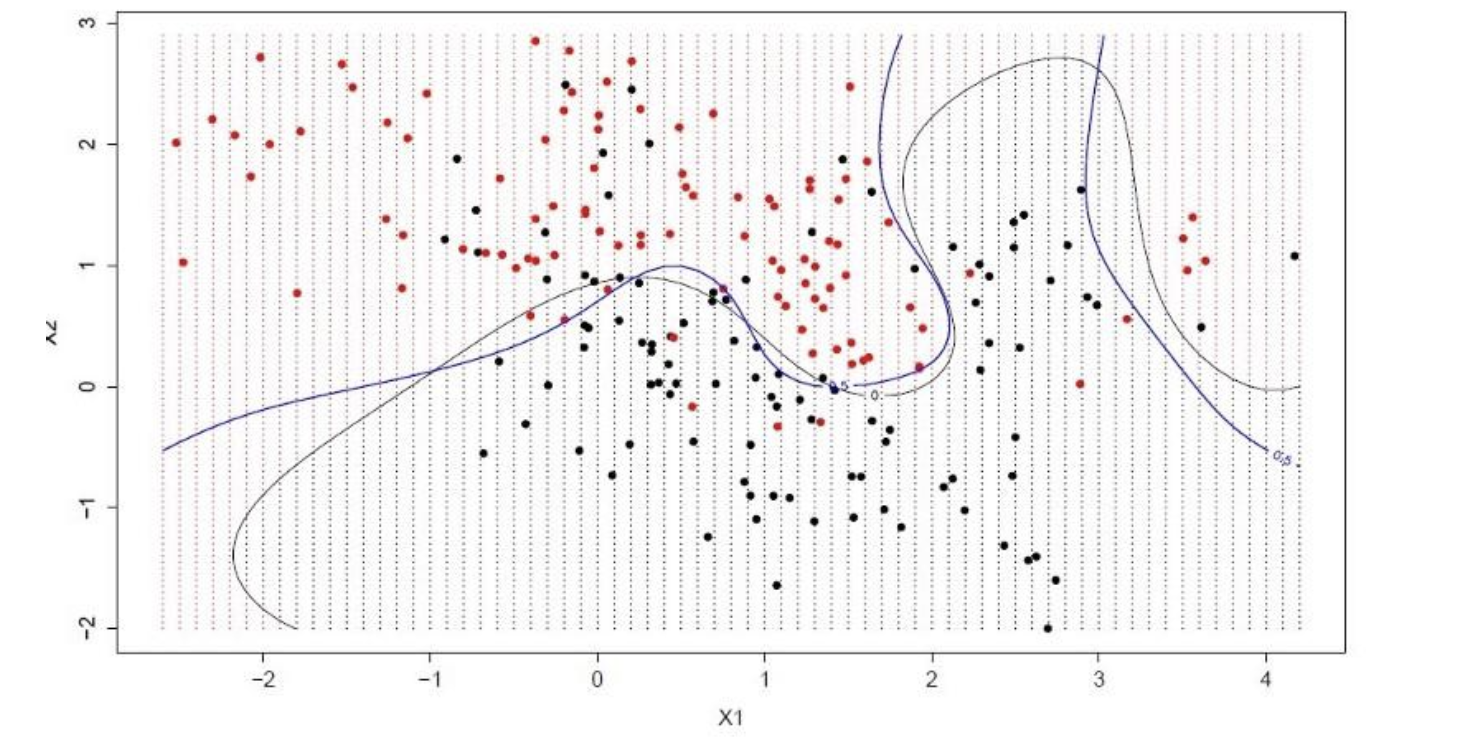## Bagging and Random Forest

Random Forest is one of the most popular and most powerful machines learning algorithms. It is a type of ensemble machine learning algorithm called Bootstrap Aggregation or bagging.

The bootstrap is a powerful statistical method for estimating a quantity from a data sample. Such as a mean. You take lots of samples of your data, calculate the mean, then average all of your mean values to give you a better estimation of the true mean value.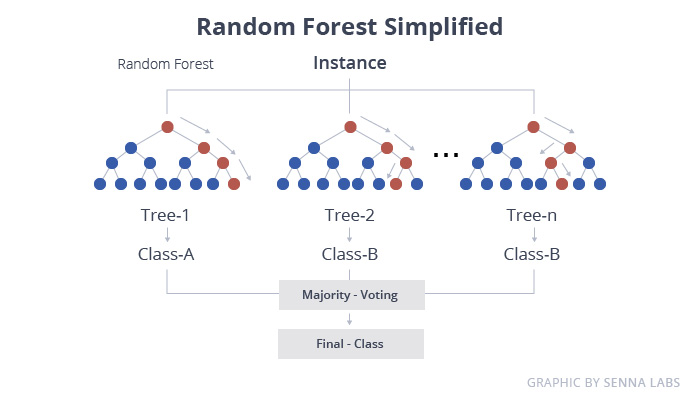## Apriori

The Apriori algorithm is used in a transactional database to mine frequent itemsets and then generate association rules. It is popularly used in market basket analysis, where one checks for combinations of products that frequently co-occur in the database. In general, we write the association rule for ‘if a person purchases item X, then he purchases item Y’ as X -> Y.

For example: if a person purchases milk and sugar, then she is likely to purchase coffee powder. This could be written in the form of an association rule as {milk, sugar} -> coffee powder. Association rules are generated after crossing the threshold for support and confidence.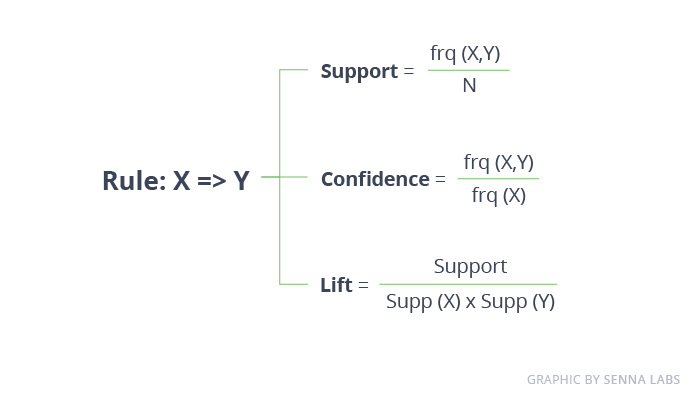## Conclusion

A typical question asked by a beginner, when facing a wide variety of machine learning algorithms, is “which algorithm should I use?” The answer to the question varies depending on many factors, including:

- The size, quality, and nature of data

- The available computational time

- The urgency of the task

- What you want to do with the data

Even an experienced data scientist cannot tell which algorithm will perform the best before trying different algorithms. Although there are many other Machine Learning algorithms, these are the most popular ones. If you’re a newbie to Machine Learning, these would be a good starting point to learn.

Read more interesting articles at Senna Labs blog

Written bySenna Labs- More than 120,000 people/day visit to read our blogs

## Other articles for you

30
September, 202330 September, 2023
Explanation of different kinds of Machine Learning models/strategies and their use cases
Last time, we mentioned how to invest a machine learning for an MVP product successfully. In this article, we will go furthermore on how to choose an appropriate machine learning

By

English
30
September, 202330 September, 2023
Choosing the appropriate machine algorithm in real use cases
In the real machine learning project, a typical question that always asked is; when facing a wide variety of machine algorithm, is "Which algorithm should we use ?" but the

By

English
30
September, 202330 September, 2023
How to successfully invest in machine learning in an MVP
A minimum viable product (MVP) is a version of a product with contains enough features to satisfy early customers and validate ideas early in the development cycle for future development.

By

English

## Let’s build digitalproductsthat aresimply awesome !

We will get back to you within 24 hours!Please tell us your ideas.
- Senna Labsmake it happy28/11 Soi Ruamrudee, Lumphini, Pathumwan, Bangkok 10330+66 62 389 4599hello@sennalabs.com© 2022 Senna Labs Co., Ltd.All rights reserved.